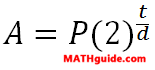Logarithms: Bacterial Growth
View the Lesson | MATHguide homepage Updated August 9th, 2021

 Formula:Given: There are 7 cells of a shigella flexneri colony.This colony has a double rate of 2.4 hours within an optimum medium. Find: Use the formula above to calculate the time it would take for the given colony to grow to 1800000 cells within an optimum medium. Calculate the solution for 't' to the nearest tenth using logarithms. Solution: t = hours